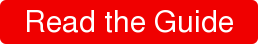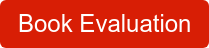# New Features of C++: Lambdas

February 8, 2018

Code written for modern C++ (C++11 and later) is often structured differently than code written for C++03 and earlier – STL algorithms are now more powerful and easier to use, more computations can be done at compile time rather than run time, writing generic code has gotten easier, and new language facilities are being used in place of older constructs.This is the first post in a series detailing language and library additions to C++ that augment existing code patterns to improve code readability, correctness, or performance over legacy strategies. While we colloquially refer to "modern" C++ as C++11 and later, in truth
modern C++ really a collection of best practices from the language's inception through the latest iteration of the standard. We start this discussion with lambdas, which were introduced in C++11.

## Lambdas

Lambdas are syntactic sugar for code you used to write by hand in C++98; namely they replace the notion of "functors", which allow you to use a callable function as a data object. For instance, if you wanted to write a function that took an arbitrary range of arithmetic values and cube the values in the range, storing the new value back over the old value, you might write a helper function that looks like:

`#include <algorithm>`
`#include <cmath>`
`#include <functional>>`

`template <typename Iter>`

`void cube(Iter begin, Iter end) {`
`  using namespace std::placeholders;`

`  std::transform(`
`        begin, end, begin,`
`      std::bind(static_cast<double (*)(double, double)>(std::pow), _1, 3.0));`

`}`

This code uses std::bind() to bind the value 3.0 as the exponent to a call to std::pow, and uses the placeholder syntax to represent the value being transformed from the iterator. The call to std::bind returns a functor that can be called by std::transform. In modern C++, you can use a lambda to replace the functor returned from std::bind(), which also removes the need to disambiguate which overload of std::pow() is being used.

`#include <algorithm>`
`#include <cmath>`

`template <typename Iter>`

`void better_cube(Iter begin, Iter end) {`

`  std::transform(begin, end, begin, [](double val) {`
`    return std::pow(val, 3.0);`
`  });`

`}`

The lambda is introduced with the lambda introducer syntax (the square brackets), and the lambda accepts a single argument (a value from the range passed to better_cube()) and it returns the cubed value (letting the compiler pick the appropriate overload rather than having to explicitly spell it out).

Lambdas are incredibly powerful – not only do they allow you to treat a function as data that can be passed around as needed, they can also capture information from their local environment to be used when the lambda is invoked. For instance, if we wanted to augment our helper function to accept an arbitrary exponent, we could "capture" the exponent value thus:

`#include <algorithm>`
`#include <cmath>`

`template <typename Iter>`

`void range_pow(Iter begin, Iter end, double exponent) {`

`  std::transform(begin, end, begin, [exponent](double val) {`
`    return std::pow(val, exponent);`
`  });`

`}`

The lambda introducer syntax allows you to "capture" values from the environment (capture by value or capture by reference) to be used when the function object is called. Values can even be captured implicitly so that you do not have to spell out the captures in the lambda introducer, but instead implicitly refer to the variable by name within the lambda. For instance, this solution is equivalent to the other solution:

`#include <algorithm>`
`#include <cmath>`

`template <typename Iter>`

`void range_pow(Iter begin, Iter end, double exponent) {`

`  std::transform(begin, end, begin, [=](double val) {`
`    return std::pow(val, exponent);`
`  });`

`}`

This isn't intended as a full lecture on lambdas, but I want to stress what a game-changer lambdas have been for writing C++ code. While they certainly make using algorithms from the STL far easier (and largely negate the need for error-prone constructs like std::bind()), their use extends to any circumstance where you might want to take a callable argument, such as thread start routines, packaged tasks for continuation-style passing, callback functions, and much more.

If you want your function to accept a lambda as an argument, you should do so by using templates because all lambdas have a unique, unnamable type associated with them. Use of a template means you don't have to care about the type of the lambda, just the functionality. This has an extra benefit of working with non-lambda objects as well! e.g.,

`#include <iostream>`

`template <typename Callable>`

`void call_this(Callable &&call) {`
`  call();`
`}`

`void func() { std::cout << "Called func" << std::endl; }`

`int main() {`

`  call_this([]() { std::cout << "Called lambda" << std::endl; });`
`  call_this(func);`

`  struct {`
`    void operator()() const { std::cout << "Called functor" << std::endl; }`
`  } s;`
`  call_this(s);`
`}`

Lambdas have changed the game for writing clean C++ code. Older code
would have to write functors manually if they needed to keep state
information while working with STL algorithms or other callback
functions. This mostly boilerplate code can now be replaced with a
concise syntax allowing you to express the same constructs.

In part 2, I'll discuss move semantics.###### Interested in trying CodeSonar or CodeSentry for yourself?### Posts by Topic

#### Related posts

##### C was Programming Language of the Year 2019By Mark Hermeling - January 21, 2020
##### An Update from the C Standards Committee WG14By Aaron Ballman - December 9, 2019
##### Merging of the MISRA C++ and AUTOSAR C++ Guidelines is Good News for Safety Critical Software DevelopmentBy Mark Hermeling - June 20, 2019